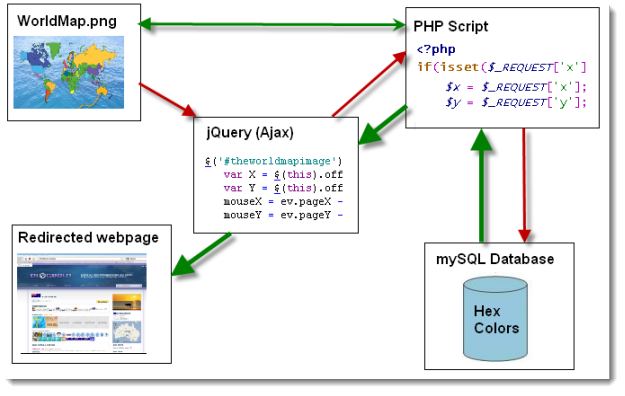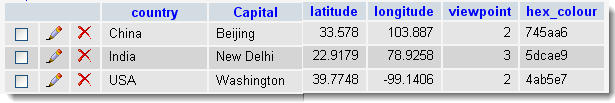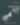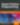# Dynamic Image Map Based on Pixel Colour

JavaScript

Share:

#### Free JavaScript Book!Write powerful, clean and maintainable JavaScript.

RRP \$11.95

So today I created a html image map which creates dynamic links based on the color of the pixel that the user clicked. The reason for creating this was because the map was a vector map of the world and as you know all countries have varying sizes and it would be impossible to create a normal point/array based image map… it would simple be too huge to store and would take too long to load.

## Pro’s of this method

• No need to know what areas of the image are covered by what for links
• Image areas can be any shape or size

## How it works1. User clicks anywhere on the image
2. jQuery captures the x and y coordinates of where the user clicked
3. x and y coordinates sent to PHP control script via Ajax
4. PHP script gets the image locally and picks the pixel colour at the x and y coordinates
5. PHP script gets the country that matches the hex colour value
6. PHP script sends back the url of the page for that country
7. jQuery redirects to the page returned via Ajax call

## Instructions on how to setup:

Create the image with unique hex values (I used adobe illustrator with a free vector based image).Store the hex colour values of the pixels contained within the boundaries of the each country in your database.jQuery Code:

``````jQuery(document).ready(function(\$) {
\$('#theworldmapimage').live('click', function(ev) {
var X = \$(this).offset().left;
var Y = \$(this).offset().top;
mouseX = ev.pageX - X;
mouseY = ev.pageY - Y;
//FIX X AXIS ERORR MARGIN
//	mouseY -= 5;
if (mouseX > 500) { mouseX -= 13; }
\$.get("../php/php-functions/phpfunc-imagemap.php",{x: mouseX ,y: mouseY}, function(data){
if (data != '') {
//alert(data + " " +"x="+mouseX+" y="+mouseY);
window.location.replace(data); //load the url of the clicked country
}
else {
}
});
});
});``````

PHP Code:

``````< ?php
if(isset(\$_REQUEST['x'])) {
\$x = \$_REQUEST['x']; \$y = \$_REQUEST['y'];
\$im = imagecreatefrompng(\$domain."/images/worldmap-coloured.png");
\$rgb = imagecolorat(\$im, \$x, \$y);
\$r = (\$rgb >> 16) & 0xFF;
\$g = (\$rgb >> 8) & 0xFF;
\$b = \$rgb & 0xFF;

function rgb2html(\$r, \$g=-1, \$b=-1)
{
if (is_array(\$r) && sizeof(\$r) == 3)
list(\$r, \$g, \$b) = \$r;
\$r = intval(\$r); \$g = intval(\$g);
\$b = intval(\$b);
\$r = dechex(\$r<0?0:(\$r>255?255:\$r));
\$g = dechex(\$g<0?0:(\$g>255?255:\$g));
\$b = dechex(\$b<0?0:(\$b>255?255:\$b));
\$color = (strlen(\$r) < 2?'0':'').\$r;
\$color .= (strlen(\$g) < 2?'0':'').\$g;
\$color .= (strlen(\$b) < 2?'0':'').\$b;
return \$color;
}

\$hex = rgb2html(\$r, \$g, \$b);
//echo ("R".\$r." G".\$g." B".\$b."  hex=#".\$hex);
\$debug = ("R".\$r." G".\$g." B".\$b."  hex=#".\$hex);
\$html = "";

\$qry = "SELECT country FROM `countries` WHERE hex_colour= '".\$hex."' LIMIT 1;";
if (mysql_query(\$qry)) {
\$result = mysql_query(\$qry);
while (\$row = mysql_fetch_array(\$result,MYSQL_ASSOC)){
\$country_filename = convertToFileName(\$row{'country'});
\$html = \$domain."/".\$country_filename."/";
\$debug .= " " . \$country_filename;
}
}
mysql_close(\$dbh);
echo \$html;
}
?>``````

The final image looks like this:See Demo (Click on the “Show world map” to show the dynamic image map, then click on any country).### Sam Deering

Sam Deering has 15+ years of programming and website development experience. He was a website consultant at Console, ABC News, Flight Centre, Sapient Nitro, and the QLD Government and runs a tech blog with over 1 million views per month. Currently, Sam is the Founder of Crypto News, Australia.

#### New books out now!Learn how Git works, and how to use it to streamline your workflow!Google, Netflix and ILM are Python users. Maybe you should too?